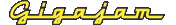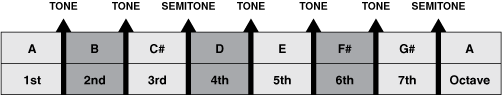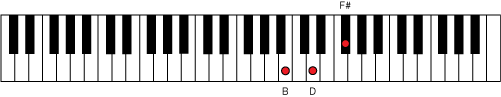EMT Lesson Three  How to create and play chords

# Minor chords

To create a chord on the second note of the scale (B), we repeat the process of creating a triad with notes which are a third apart.

In this case, take the second, fourth and sixth notes of the A major scale above. Therefore, the first note of the new chord is B, the second note is D and the third note is F#.

Figure 3. The chord of B minor   (Enlarge)Referring to the keyboard you can see that the note of D is 3 semitones above the B. This interval is called a minor third. This is what creates the B minor chord. Therefore, B + D + F# gives the chord of B minor.

Figure 4. B minor triad   (Enlarge)The difference between a major chord and a minor chord is the middle note of the triad. If the interval between the first note and the middle note is a major third (two tones) the chord is major. If this interval is a minor third (three semitones) then the chord is minor.

A very common way of referring to the construction of these chords is:

Major chord: 1, 3, 5
Minor chord: 1, b3, 5

In this terminology, the ‘1’ refers to the first note in each chord – not its place in the scale. This is because chords can be built on any note of the scale, not just the first note, as we have demonstrated above with the A major and B minor chords.